## Friday, June 8, 2018

### [XLA 研究] Take a glance to see the graph changes in XLA JIT compilation

In the preamble of this article, to understand XLA JIT is pretty hard because you probably need to understand TensorFlow Graph, Executor,  LLVM, and math... I have been through this painful study work somehow so that I hope my experience can help for those who are interested in XLA but have not get understood yet.

First, I use the following code to build my TF graph.
```W = tf.get_variable(shape=[], name='weights')
b = tf.get_variable(shape=[], name='bias')
x_observed = tf.placeholder(shape=[None],
dtype=tf.float32,
name='x_observed')
y_pred = W * x_observed + b
learning_rate = 0.025

y_observed = tf.placeholder(shape=[None], dtype=tf.float32, name='y_observed')

loss_op = tf.reduce_mean(tf.square(y_pred - y_observed))
train_op = optimizer_op.minimize(loss_op)```
I try to dump all the temporary graphs during the XLA JIT compilation by the following flags and env variable. So, after execution, you will get a lot of pbtxt files and log.
```export TF_CPP_MIN_VLOG_LEVEL=2
TF_XLA_FLAGS="--xla_hlo_profile --tf_xla_clustering_debug --tf_dump_graph_prefix=/tmp --vmodule=xla_compiler=2 --xla_hlo_graph_path=/tmp --xla_generate_hlo_graph=.*" python test.py 2>&1 | tee xla_log.txt
```
Unfortunately, protobuf library cannot read some of the XLA dump pbtxt files so that I only can show what I get.
The way to convert pbtxt file to PNG image is here for reference:
```import tensorflow as tf
import sys

from google.protobuf import text_format
from graphviz import Digraph

dot = Digraph()

if len(sys.argv) != 2:
sys.exit("convert <graphdef.pb>")

with tf.gfile.FastGFile(sys.argv, 'rb') as f:
graph_def = tf.GraphDef()
tf.import_graph_def(graph_def)

for n in graph_def.node:
dot.node(n.name, label=n.name)

for i in n.input:
# Edges are determined by the names of the nodes
dot.edge(i, n.name)

dot.format = 'png'
dot.render(sys.argv + ".gv", view=True)```

So, based on my graph generated at the beginning of the article, we only need focus on these two TensorFlow subgraphs:
1. W = tf.get_variable(shape=[], name='weights')
2. b = tf.get_variable(shape=[], name='bias')

The reason why we only to see these is that the following explanation about XLA JIT Compilation is all around them.
Actually, XLA JIT compilation process is very complicated. I only want to list the process items of XLA JIT Compilation in execution period.

Before going into HLO optimization phase, TensorFlow will do JIT Compilation Pass first, which contains 3 main steps:

1. Mark For Compilation Pass:

2. Encapsulate Subgraph Pass:

3. Build Xla Launch Ops Pass:

Then, before rendering low-level assembly code for the graph, TensorFlow will run HLO Pass Pipeline Optimization using HLO IR, which contains several steps:

1. Optimization:2. Simplification: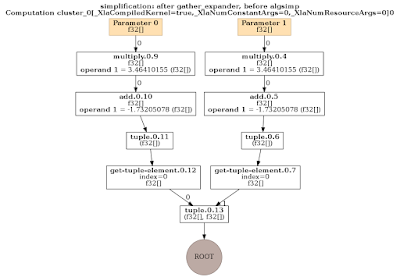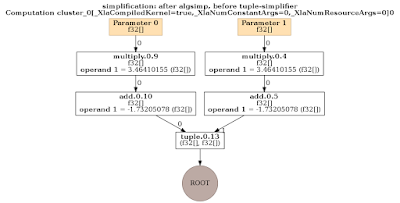3. Conv Canonicalization:4. Layout Assignment: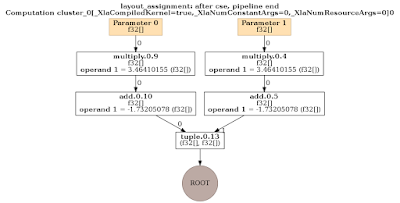5. Fusion: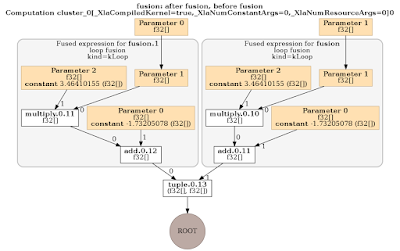6. Reduce Precision: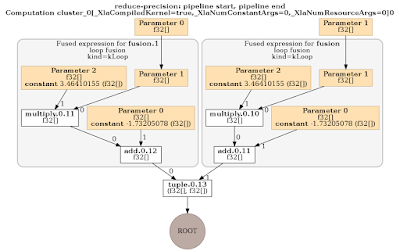7. GPU IR Emit Prepare: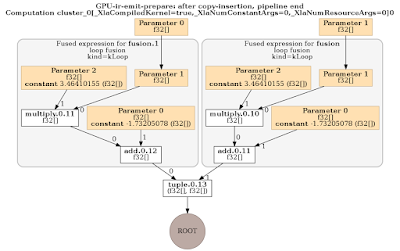The HLO IR looks like this:The LLVM IR looks like this: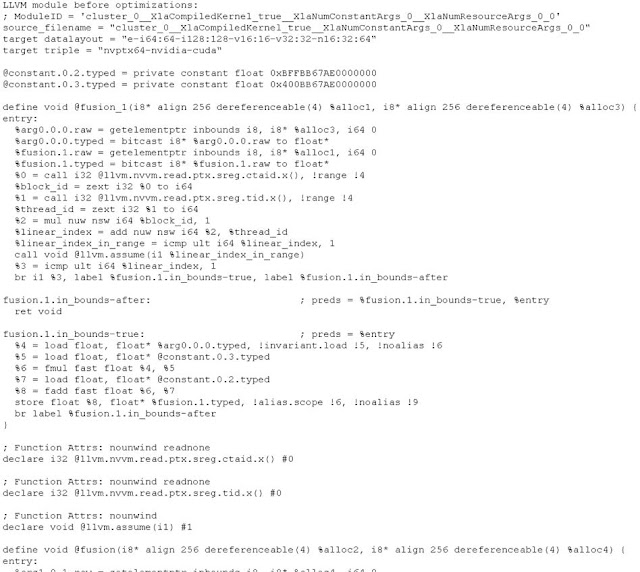In this phase, XLA can use GPU Compiler to run backend for generating binary executable code based on LLVM IR, but this is not in my discussion this time.